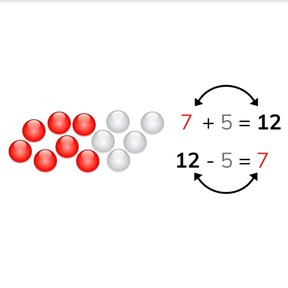Subtraction to 20 via inverse operation

# Subtraction to 20 via inverse operation

No account needed.8,000 schools use Gynzy92,000 teachers use Gynzy1,600,000 students use Gynzy

## General

Students learn to subtract numbers to 20 using the inverse operation. They learn that each subtraction number has a partner addition problem, which they can use to solve the subtraction problem.

1.OA.C

## Relevance

This strategy helps to make subtraction easier and faster. It helps students better understand calculations to 20.

## Introduction

Start by decomposing the numbers given on the interactive whiteboard. Then discuss the number bonds for the numbers given on the interactive whiteboard. You may also encourage students to use blocks to help represent these decompositions/number bonds. Do a few number decompositions orally with the class. For example: " I decompose the number 15 into 4 and ...". Then determine which math problems are shown in the images on the interactive whiteboard. You see 16 apples in total, of which 3 are on the ground. This could represent 16 - 3 or 13 + 3. Discuss with students why the other options are incorrect (for example 13 - 3 is incorrect, because the total amount of apples is 16 and not 13).

## Development

Explain to students that if they know an addition problem, that they also know the subtraction problem. The numbers in the problems are simply in different places in the problem. With a subtraction problem, you start with the total or sum of the addition problem. If you subtract or take away one of the numbers, your difference is the second number. Show this with the beads on the interactive whiteboard. Then practice this with a new set of beads. Then show a decomposition of the number 5 and the math problems you can create with these decompositions. Practice this with students using different decompositions. After practicing this with students using numbers that have visual support, explain that this does not change, even if you can't count the object(s). Practice using the inverse operation with decompositions where only numbers are visible.

Check that students understand subtraction to 10 via the inverse operation by asking the following questions:
- The number 18 can be decomposed into 12 and ...?
- 8 + 9 = 17, what is 17 - 8?

## Guided practice

Students are first given a subtraction problem with visual support in the form of beads and with the addition problem given. Then they are given the decomposed number but must fill in both the subtraction problem and the inverse. Finally students must determine the subtraction problem and the inverse from numbers only.

## Closing

Discuss with students that it is important to be able to do this, so that you can calculate easier and quicker. It is useful to be able to determine, if there are 13 apples in the tree and 1 on the ground, that the total is 14. You then also know that if there are 14 apples in total and 1 is on the ground, that you still have 13 apples in the tree. To close first do some subtraction problems with visual support and end with subtraction problems without visual support.

## Teaching tips

Students who have difficulty with this can set out the math problems with MAB blocks or on the rekenrek. Have them decompose 18 into 13 and 5. Have them create the addition problem (13 + 5 = 18) and then show them that if they move the blocks around they can also create the subtraction problem(s) (18 - 13 = 5 or 18 - 5 = 13). Emphasize that they don't need to count the blocks again, because they already know which numbers are in the problems.

## Instruction materials

optional: MAB blocks or rekenrek

### The online teaching platform for interactive whiteboards and displays in schools

• Save time building lessons

• Manage the classroom more efficiently

• Increase student engagement